# Electricity and Magnetism 1 Static electricity 2 Electric

• Slides: 36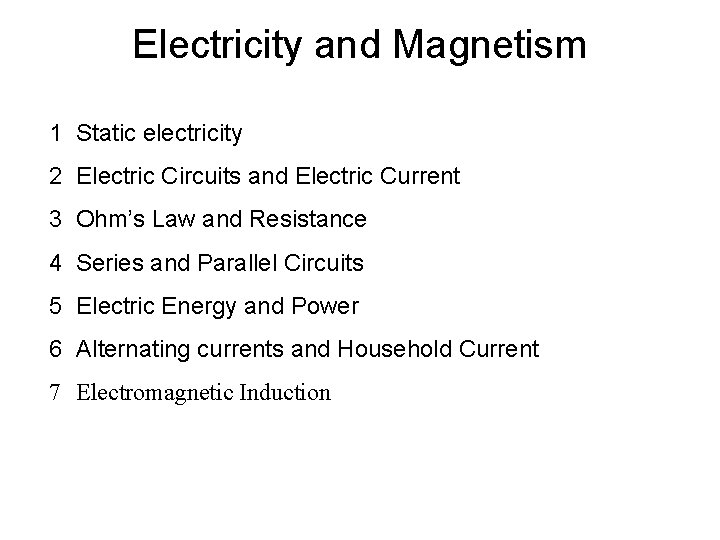Electricity and Magnetism 1 Static electricity 2 Electric Circuits and Electric Current 3 Ohm’s Law and Resistance 4 Series and Parallel Circuits 5 Electric Energy and Power 6 Alternating currents and Household Current 7 Electromagnetic InductionLightning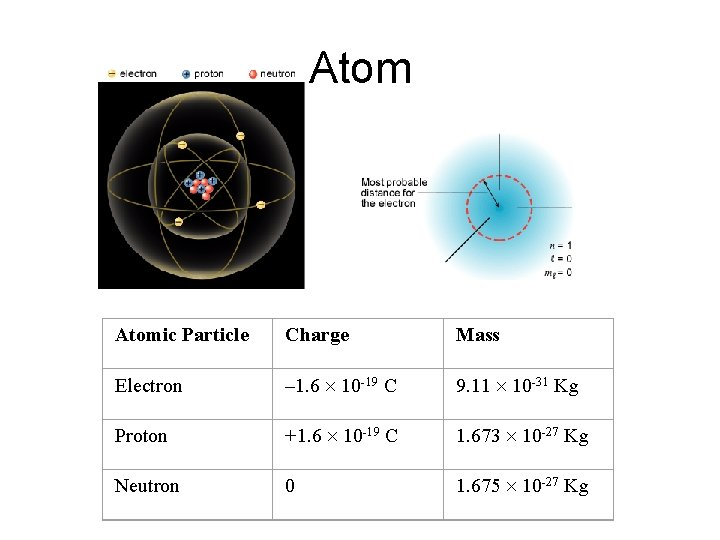Atomic Particle Charge Mass Electron – 1. 6 10 -19 C 9. 11 10 -31 Kg Proton +1. 6 10 -19 C 1. 673 10 -27 Kg Neutron 0 1. 675 10 -27 Kg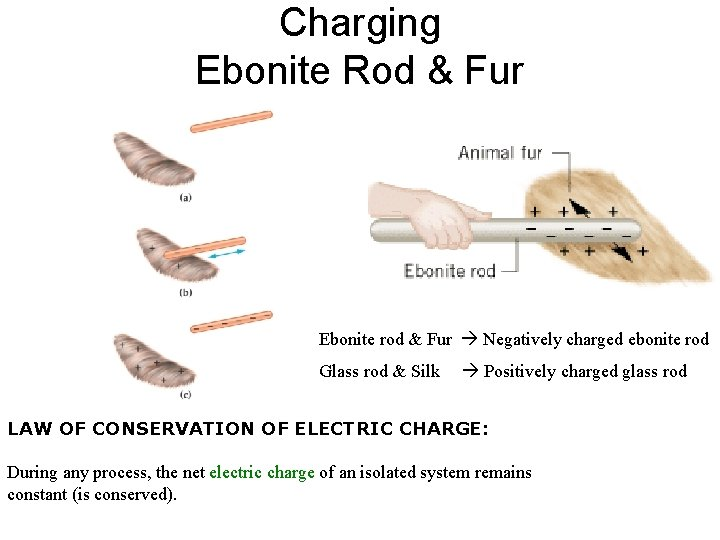Charging Ebonite Rod & Fur Ebonite rod & Fur Negatively charged ebonite rod Glass rod & Silk Positively charged glass rod LAW OF CONSERVATION OF ELECTRIC CHARGE: During any process, the net electric charge of an isolated system remains constant (is conserved).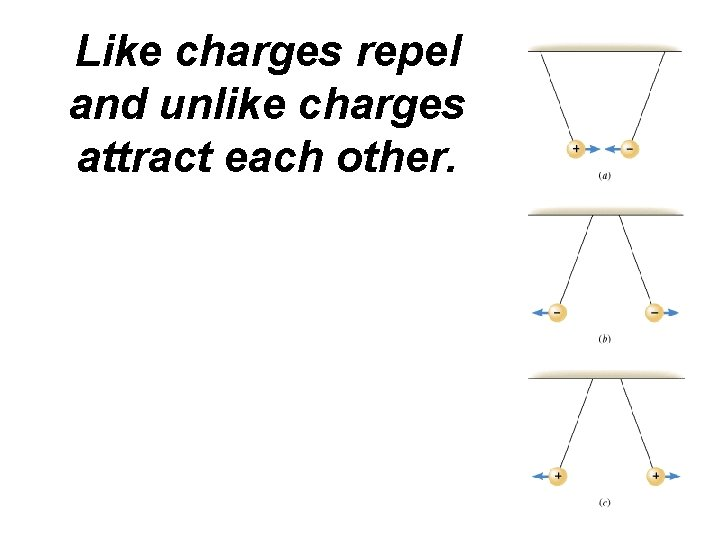Like charges repel and unlike charges attract each other.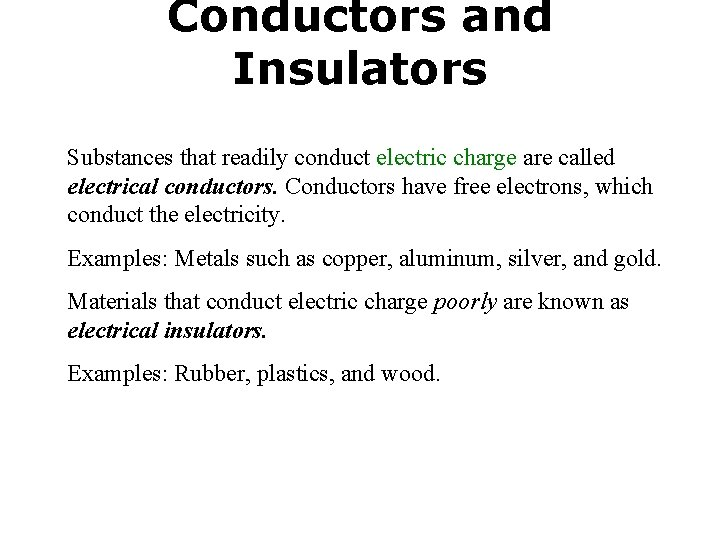Conductors and Insulators Substances that readily conduct electric charge are called electrical conductors. Conductors have free electrons, which conduct the electricity. Examples: Metals such as copper, aluminum, silver, and gold. Materials that conduct electric charge poorly are known as electrical insulators. Examples: Rubber, plastics, and wood.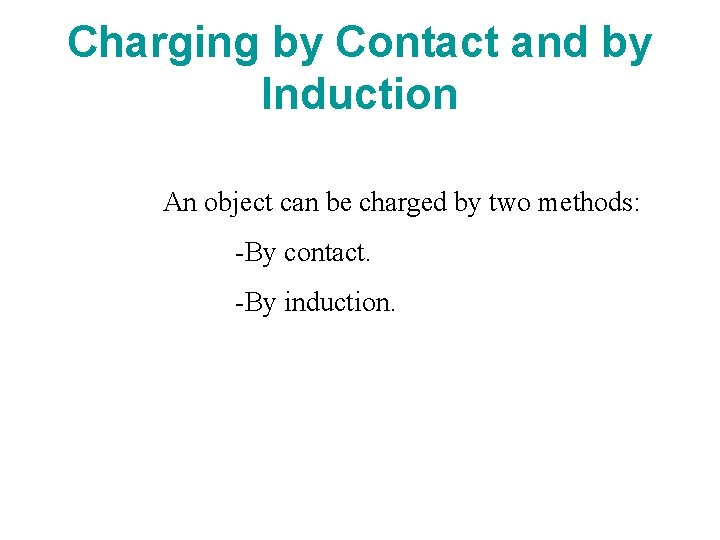Charging by Contact and by Induction An object can be charged by two methods: -By contact. -By induction.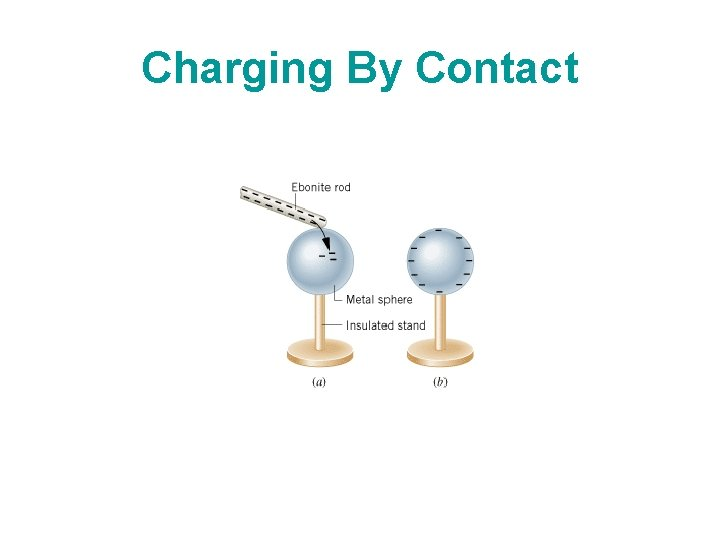Charging By ContactCharging By Induction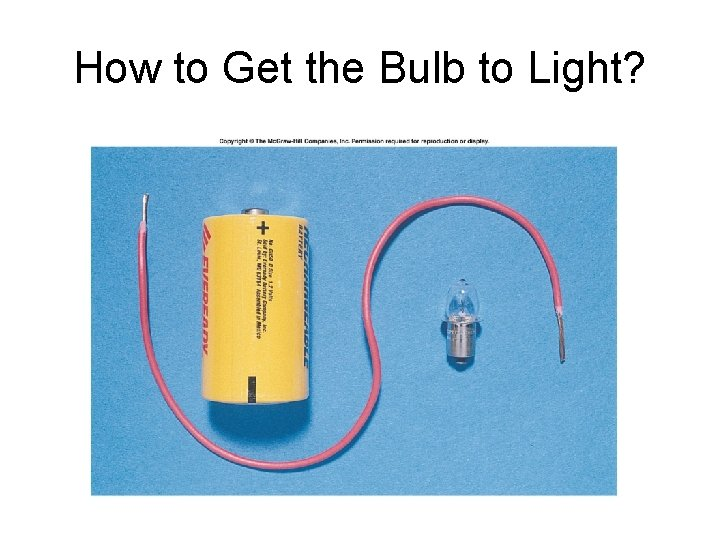How to Get the Bulb to Light?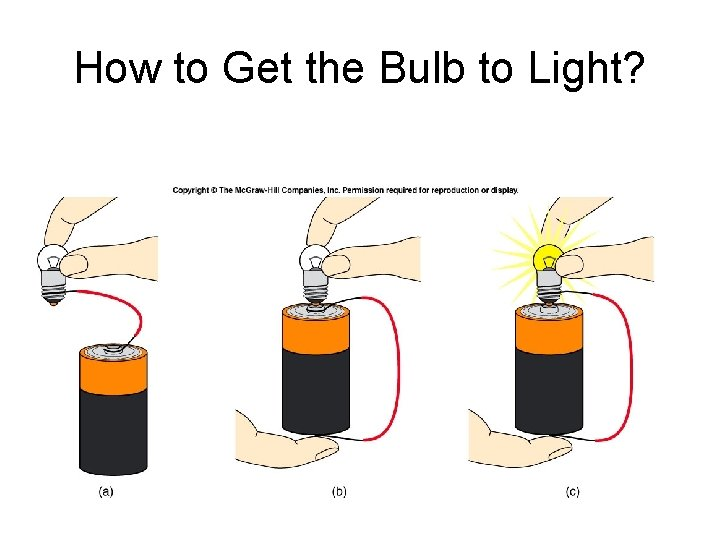How to Get the Bulb to Light?Electric Current The electric current is the amount of charge per unit time that passes through a surface that is perpendicular to the motion of the charges. The SI unit of electric current is the ampere (A), after the French mathematician André Ampére (1775 -1836). 1 A = 1 C/s. Ampere is a large unit for current. In practice milliampere (m. A) and microampere (μA) are used.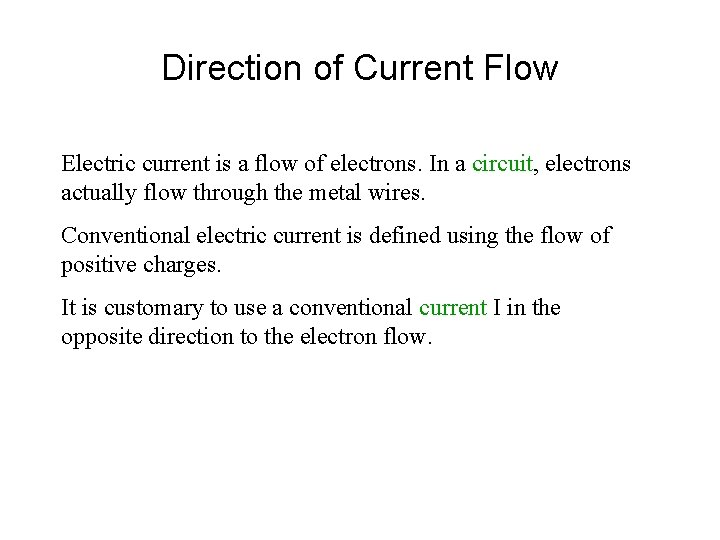Direction of Current Flow Electric current is a flow of electrons. In a circuit, electrons actually flow through the metal wires. Conventional electric current is defined using the flow of positive charges. It is customary to use a conventional current I in the opposite direction to the electron flow.Direction of Current Flow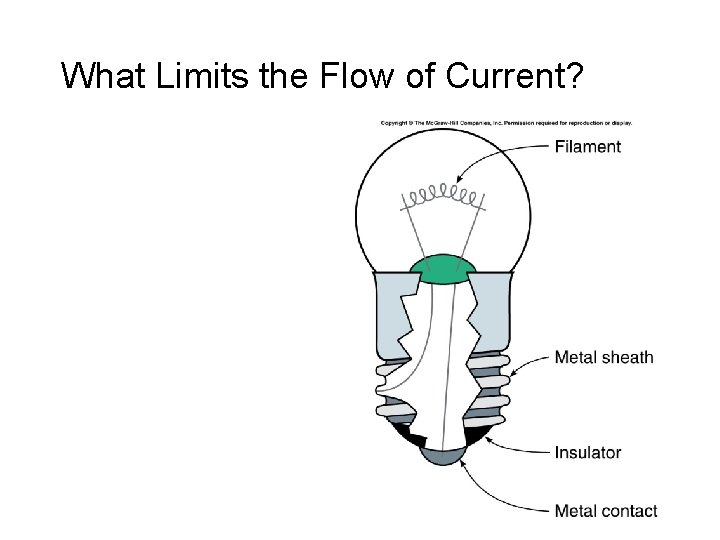What Limits the Flow of Current?What Limits the Flow of Current? A: Resistance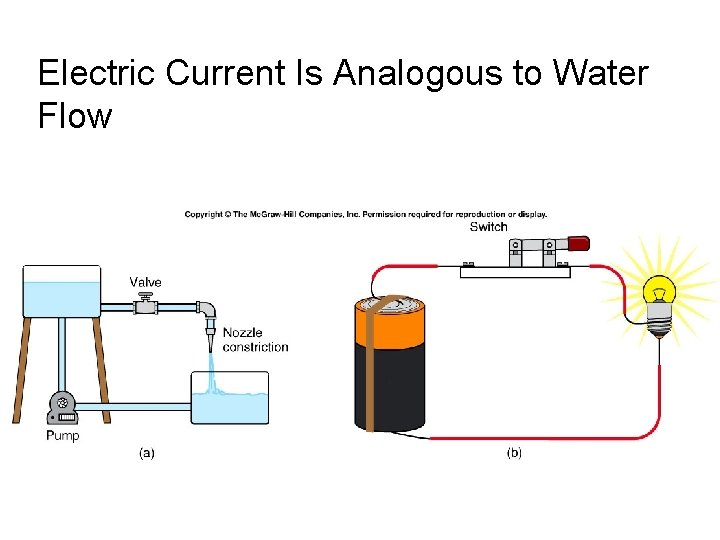Electric Current Is Analogous to Water FlowOhm’s Law Georg Simon Ohm (1787 -1854), a German physicist, discovered Ohm’s law in 1826. This is an experimental law, valid for both alternating current (ac) and direct current (dc) circuits. When you pass an electric current (I) through a resistance (R) there will be a potential difference or voltage (V) created across the resistance. Ohm’s law gives a relationship between the voltage (V), current (I), and resistance (R) as follows: V=IR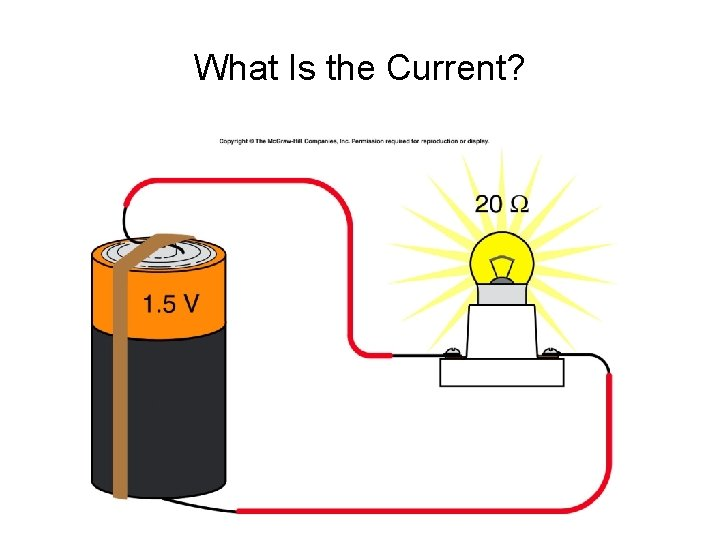What Is the Current?Electromotive Force (emf) The energy needed to run electrical devices comes from batteries. Within a battery, a chemical reaction occurs that transfers electrons from one terminal (leaving it positively charged) to another terminal (leaving it negatively charged). Because of the positive and negative charges on the battery terminals, an electric potential difference exists between them. The maximum potential difference is called the electromotive force* (emf) of the battery. The electric potential difference is also known as the voltage, V. The SI unit for voltage is the volt, after Alessandro Volta (17451827) who invented the electric battery. 1 volt = 1 J/C.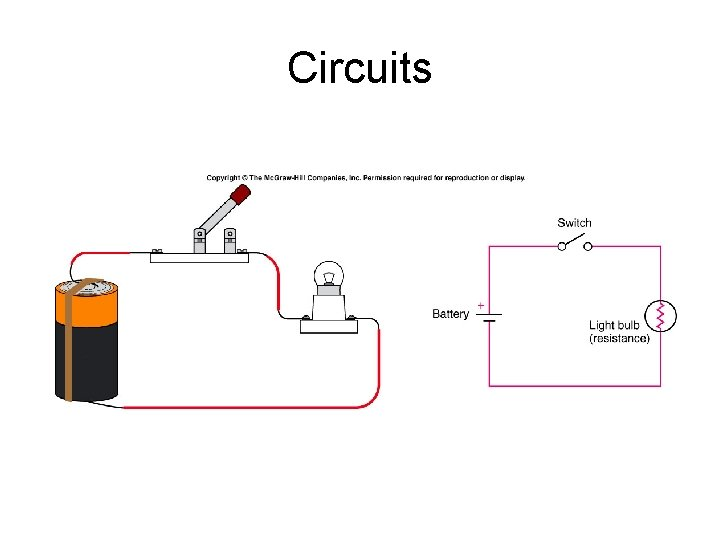CircuitsSeries CircuitParallel Circuit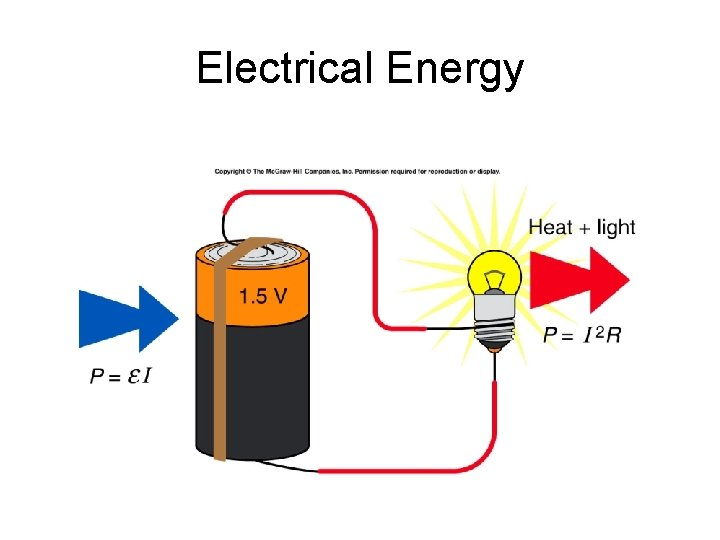Electrical EnergyElectrical Energy and Power Our daily life depends on electrical energy. We use many electrical devices that transform electrical energy into other forms of energy. For example, a light bulb transforms electrical energy into light and heat. Electrical devices have various power requirements. Electrical power, P is defined as the electrical energy transfer per unit time,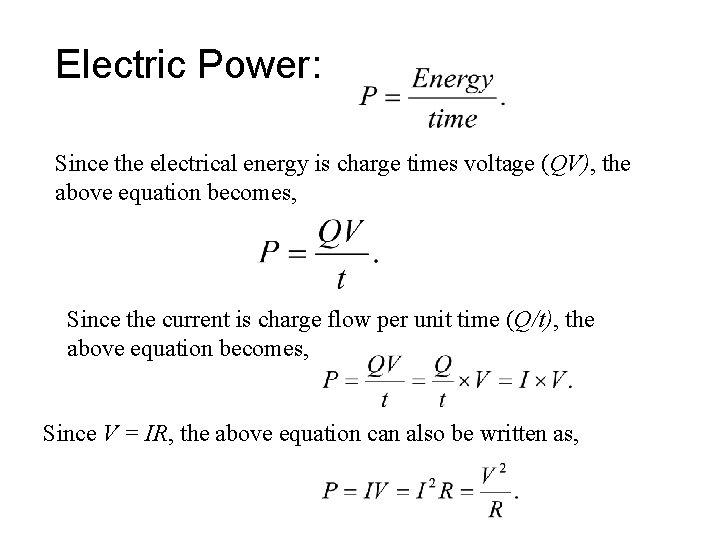Electric Power: Since the electrical energy is charge times voltage (QV), the above equation becomes, Since the current is charge flow per unit time (Q/t), the above equation becomes, Since V = IR, the above equation can also be written as,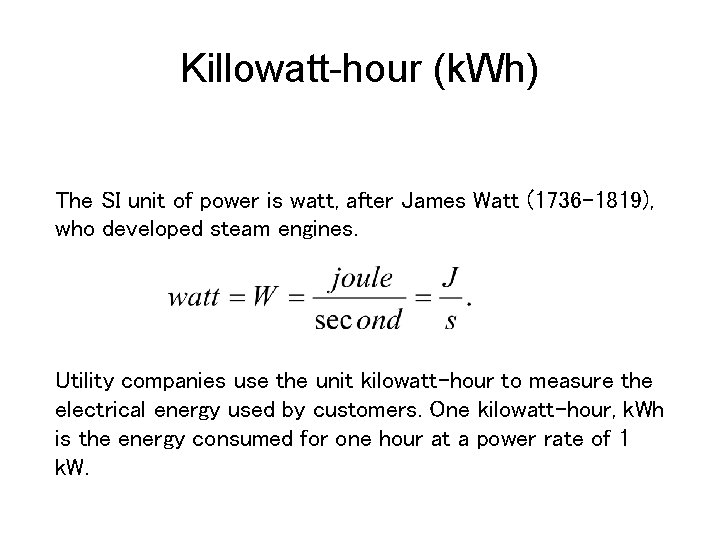Killowatt-hour (k. Wh) The SI unit of power is watt, after James Watt (1736 -1819), who developed steam engines. Utility companies use the unit kilowatt-hour to measure the electrical energy used by customers. One kilowatt-hour, k. Wh is the energy consumed for one hour at a power rate of 1 k. W.Exercises 1. State Ohm’s law in an equation form in terms of voltage and current. 2. Define power in an equation form in terms of voltage and current. 3. When an appliance is plugged in a 120 -volt outlet, it draws a current of 8 amperes. Calculate the power of the appliance. 4. If the above appliance is used 10 hours a day for 28 days per month, and if the cost of electricity is 12 cents per kilowatt‑hour, how much does it cost to operate the appliance for a year?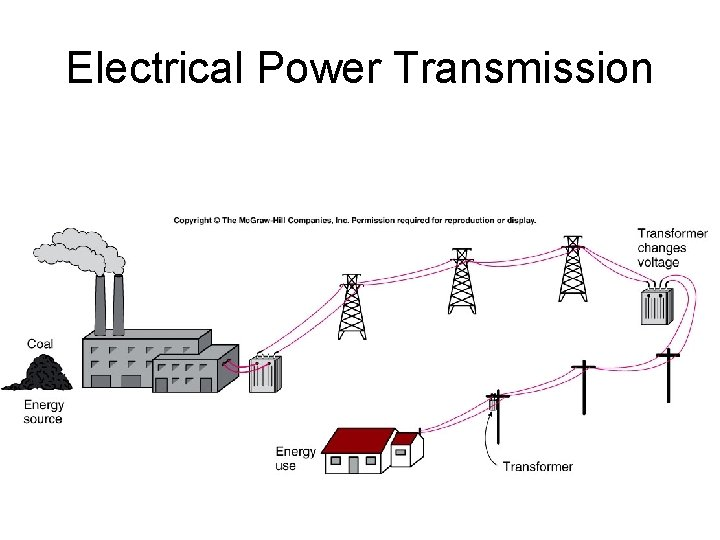Electrical Power Transmission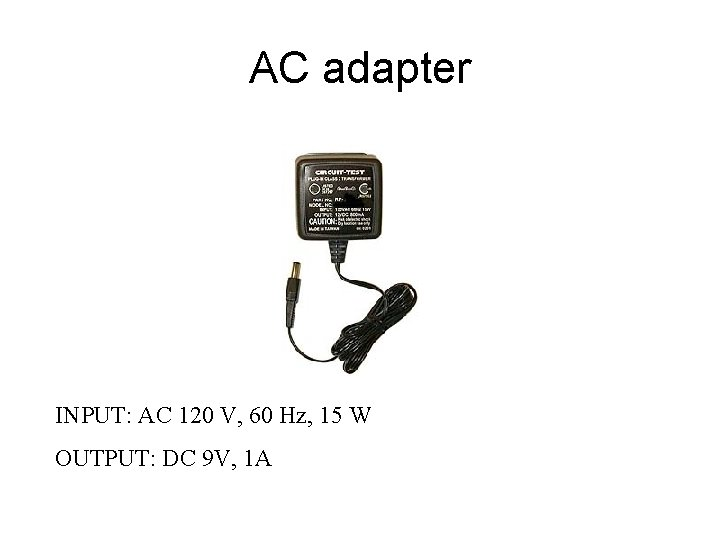AC adapter INPUT: AC 120 V, 60 Hz, 15 W OUTPUT: DC 9 V, 1 AAlternating Current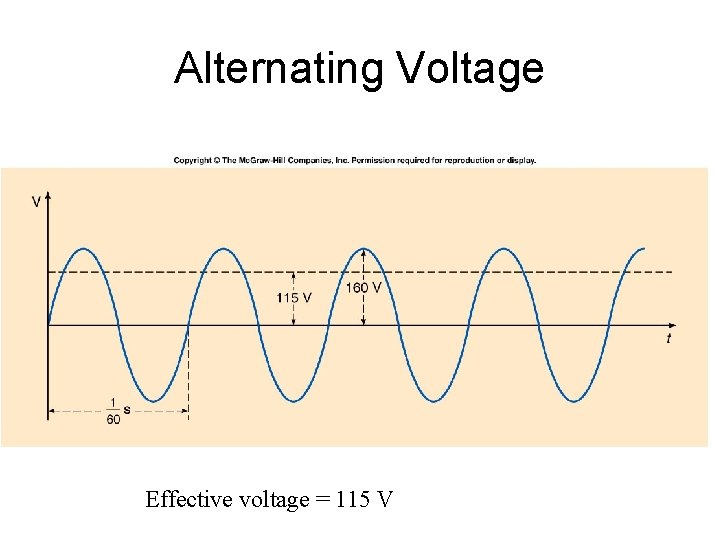Alternating Voltage Effective voltage = 115 V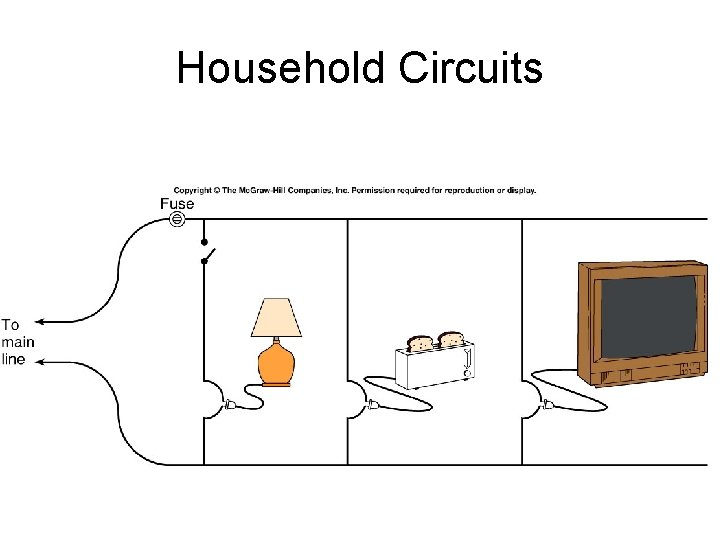Household Circuits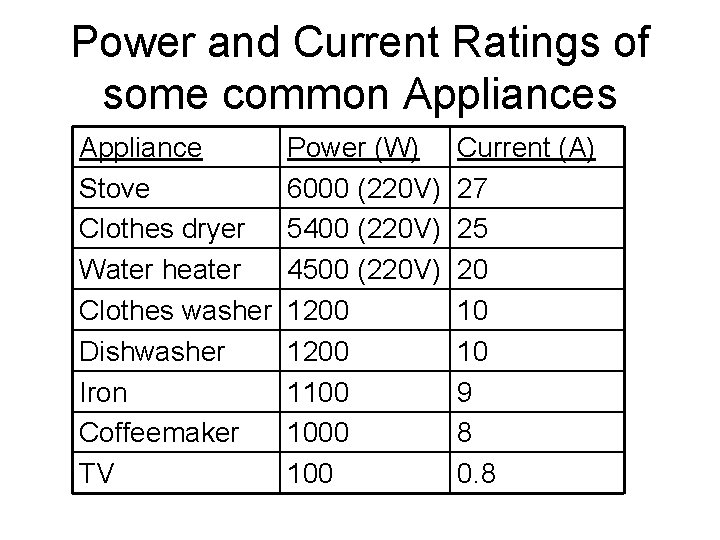Power and Current Ratings of some common Appliances Appliance Stove Clothes dryer Water heater Clothes washer Dishwasher Iron Coffeemaker TV Power (W) 6000 (220 V) 5400 (220 V) 4500 (220 V) 1200 1100 100 Current (A) 27 25 20 10 10 9 8 0. 8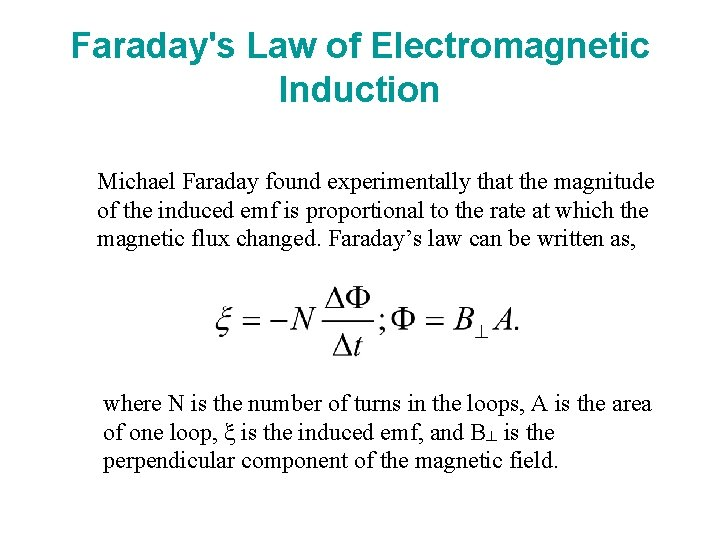Faraday's Law of Electromagnetic Induction Michael Faraday found experimentally that the magnitude of the induced emf is proportional to the rate at which the magnetic flux changed. Faraday’s law can be written as, where N is the number of turns in the loops, A is the area of one loop, ξ is the induced emf, and B┴ is the perpendicular component of the magnetic field.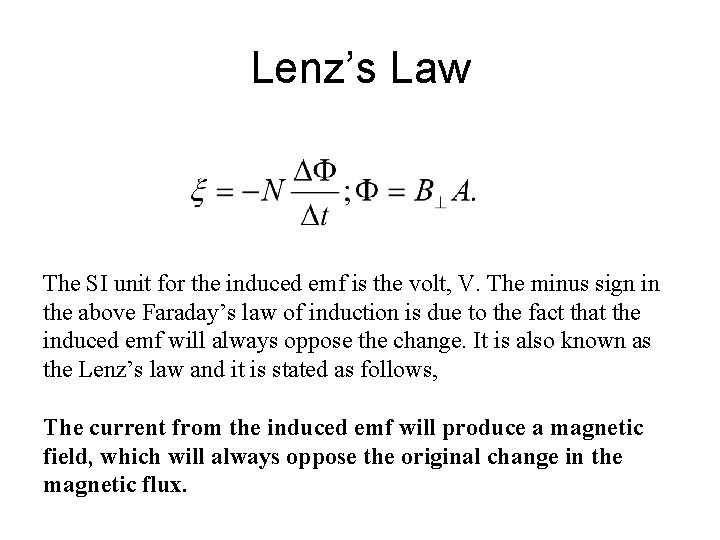Lenz’s Law The SI unit for the induced emf is the volt, V. The minus sign in the above Faraday’s law of induction is due to the fact that the induced emf will always oppose the change. It is also known as the Lenz’s law and it is stated as follows, The current from the induced emf will produce a magnetic field, which will always oppose the original change in the magnetic flux.